Like   Tweet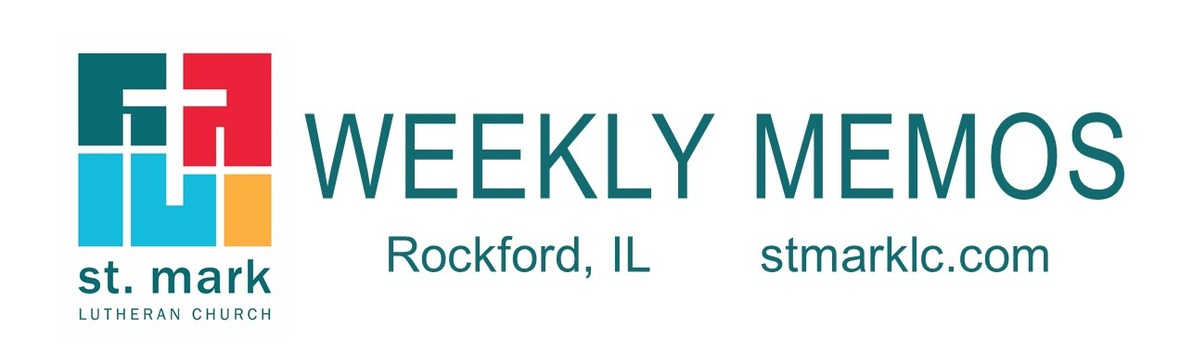table div table+table div table{width:100%;padding:0}table div table+table div table img{width:96.23%;padding:0;float:none}table div table+table div table td{width:100%;padding:0 1.88% 18px}/* styles */## Sunday, May 5

8:00 A.M. Worship
9:30 A.M. Worship
9:30 A.M. Last Day of Sunday School
11:00 A.M. Worship

## Monday, May 6

9:00 A.M. Classic Chorale Rehearses
5:00 P.M. Children's Ministry Meeting
6:30 P.M. Emotions Anonymous
6:30 P.M. Blue Lights

## Tuesday, May 7

10:00 A.M. Staff Meeting
11:00 A.M. St. Mark Duplicate Bridge
1:00 P.M. First Tuesday Book Club

## Wednesday, May 8

9:00 A.M. Women's Bible Study
9:00 A.M. Men's Bible Study
9:30 A.M. St. Mark Ringers
12:30 P.M. Miriam Circle
4:30 P.M. Outreach Ministry
5:30 P.M. Good News Bearers
6:15 P.M. Bible Study
6:30 P.M. Confirmation
7:00 P.M. Voices of St. Mark

## Thursday, May 9

7:30 A.M. Panera Book Study
9:00 A.M. Cherry Valley Bridge Group
9:30 A.M. Ruth Circle
1:00 P.M. Sew and Sews
1:00 P.M. Rebecca Circle
5:30 P.M. 20:30 Group
6:30 P.M. Emotions Anonymous
6:30 P.M. Diakonia
7:00 P.M. Praise Band Rehearses

## Friday, May 10

7:30 A.M. St. Mark Men's Breakfast at Denny's
9:30 A.M. Rod Bjerke Sr. Visitation
11:00 A.M. Rod Bjerke Sr. Memorial Service

## Saturday, May 11

8:30 A.M. Council Retreat
10:00 A.M. Family Kite Flying Day at Saturn Park
6:00 P.M. Train Group

## Sunday, May 12 Mother's Day

8:00 A.M. Worship
9:30 A.M. Worship
9:30 A.M. Teacher Recognition and Sunday School Awards
11:00 A.M. Worship

 table div table+table+table+table+table+table div table{width:100%;padding:0}table div table+table+table+table+table+table div table img{width:96.23%;padding:0;float:none}table div table+table+table+table+table+table div table td{width:100%;padding:0 1.88% 18px}/* styles */## News and Events

 table div table+table+table+table+table+table+table+table div table{width:100%;padding:0}table div table+table+table+table+table+table+table+table div table img{width:96.23%;padding:0;float:none}table div table+table+table+table+table+table+table+table div table td{width:100%;padding:0 1.88% 18px}/* styles */Welcome Lutheran High School Orchestra
St. Mark welcomes the orchestra from Rockford Lutheran High School this Sunday, May 5, under the direction of Monica Benolkin. They will be playing the Prelude, Postlude and Special music during the Offering for our 9:30 a.m. service. Thank you Rockford Lutheran, for sharing your talents in St. Mark’s worship!

 table div table+table+table+table+table+table+table+table+table+table div table{width:100%;padding:0}table div table+table+table+table+table+table+table+table+table+table div table img{width:96.23%;padding:0;float:none}table div table+table+table+table+table+table+table+table+table+table div table td{width:100%;padding:0 1.88% 18px}/* styles */Registration is now open for Vacation Bible School 2019
Volunteer for VBS too at the link below!

To Mars and Beyond: Explore Where God’s Power Can Take You!

Our amazing space adventures will take place from 9 am-noon on June 10-14. VBS is open to elementary-age students and includes daily snacks, crafts, science, games, music, Bible stories, and more! Register in person at St. Mark or online at myvbs.org/stmarklc.
Let’s explore God’s universe together!

 table div table+table+table+table+table+table+table+table+table+table+table+table div table{width:100%;padding:0}table div table+table+table+table+table+table+table+table+table+table+table+table div table img{width:96.23%;padding:0;float:none}table div table+table+table+table+table+table+table+table+table+table+table+table div table td{width:100%;padding:0 1.88% 18px}/* styles */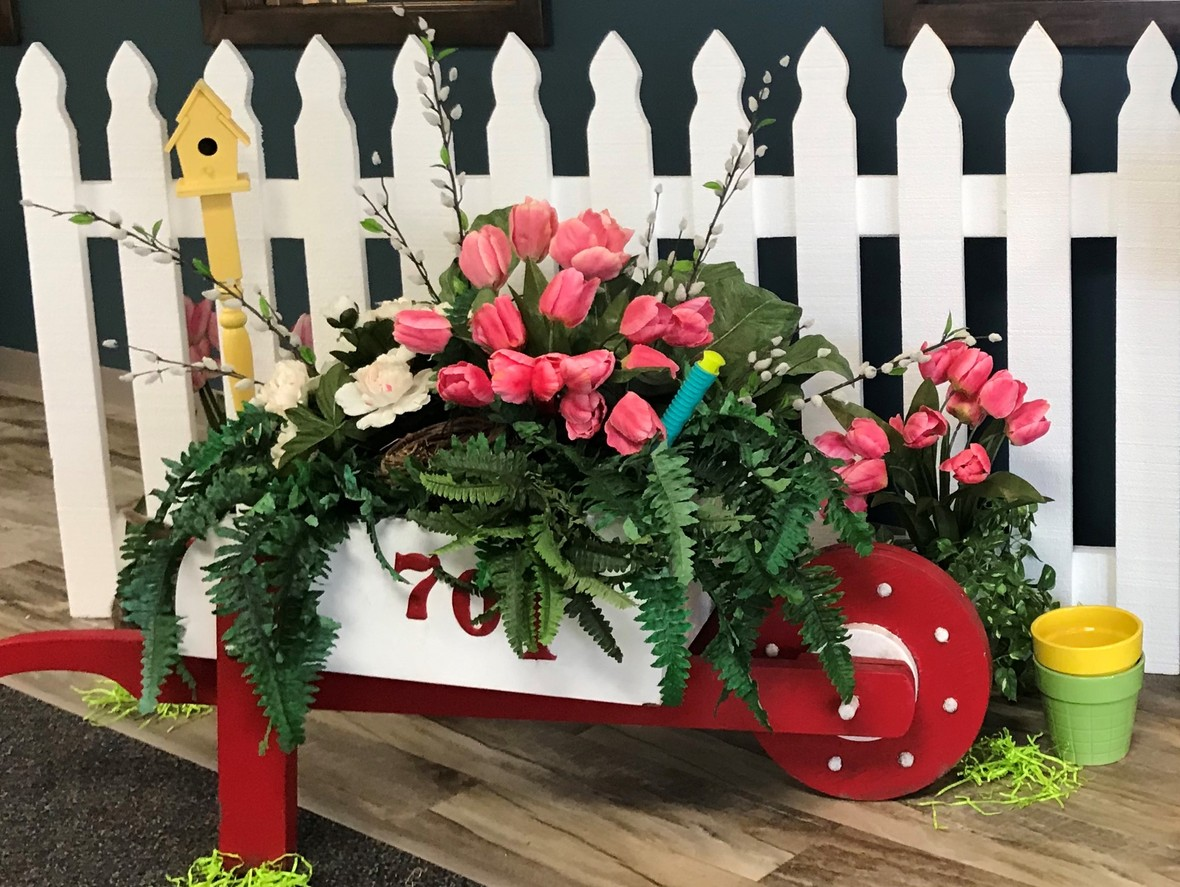Ladies “spring” event happened on Saturday, April 27.
The ladies brought “spring” into the St. Mark café, even though it was snowing outside! The fifty women that attended had fun, time for fellowship, and a tasty brunch! We even had a wonderful surprise visit from a trio of men who sang for us. It was beautiful!

One of the ladies said, “the attention to all the little details was outstanding!” The event made her feel “special.” Ladies, you are ALL special! Thank you to all that participated and to the ladies that put together a great event. Thank you!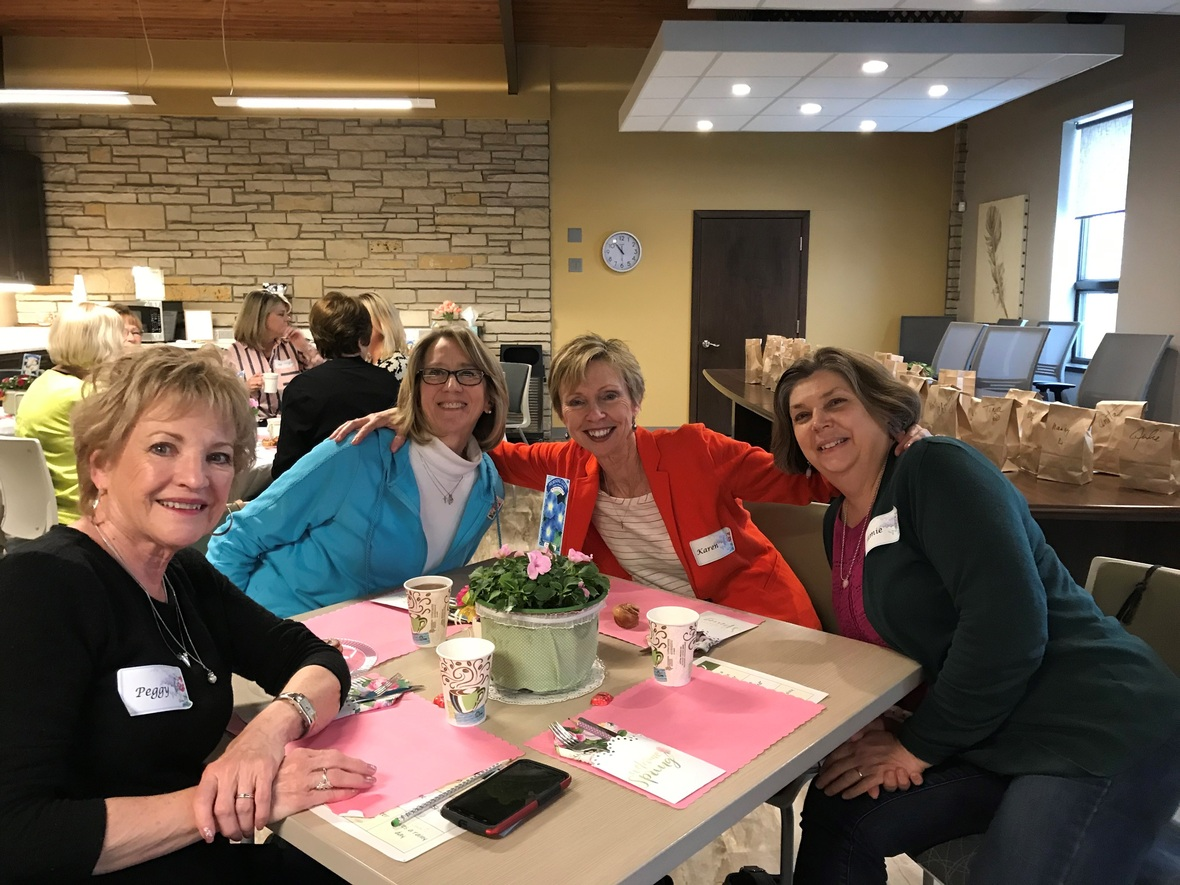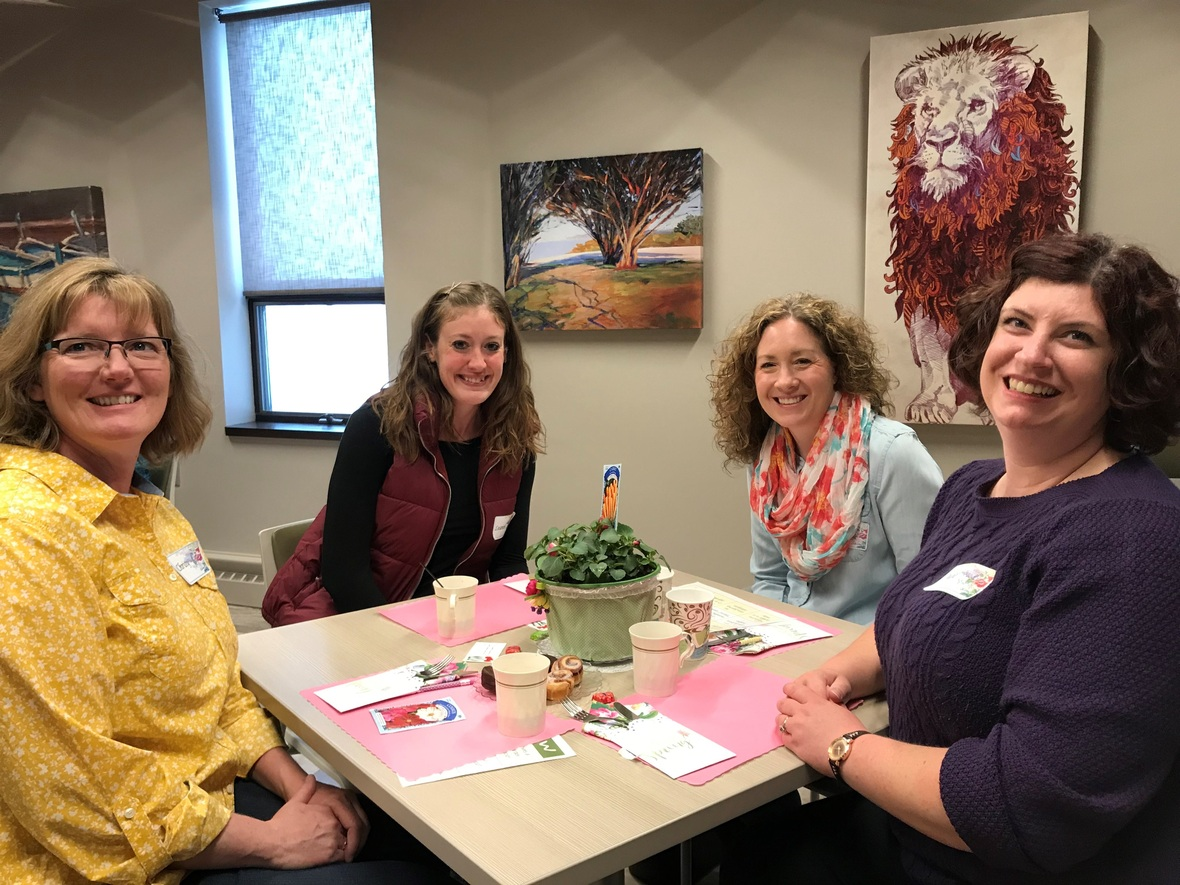table div table+table+table+table+table+table+table+table+table+table+table+table+table+table+table+table div table{width:100%;padding:0}table div table+table+table+table+table+table+table+table+table+table+table+table+table+table+table+table div table img{width:96.23%;padding:0;float:none}table div table+table+table+table+table+table+table+table+table+table+table+table+table+table+table+table div table td{width:100%;padding:0 1.88% 18px}/* styles */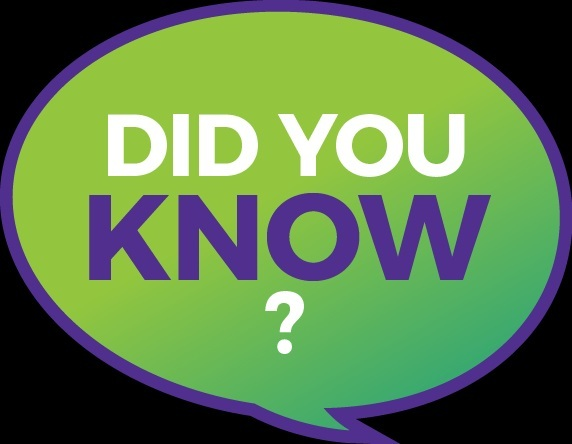Miss a Sunday at St. Mark? Did you know that you can watch the sermons online? Click here to watch.

 table div table+table+table+table+table+table+table+table+table+table+table+table+table+table+table+table+table+table div table{width:100%;padding:0}table div table+table+table+table+table+table+table+table+table+table+table+table+table+table+table+table+table+table div table img{width:96.23%;padding:0;float:none}table div table+table+table+table+table+table+table+table+table+table+table+table+table+table+table+table+table+table div table td{width:100%;padding:0 1.88% 18px}/* styles */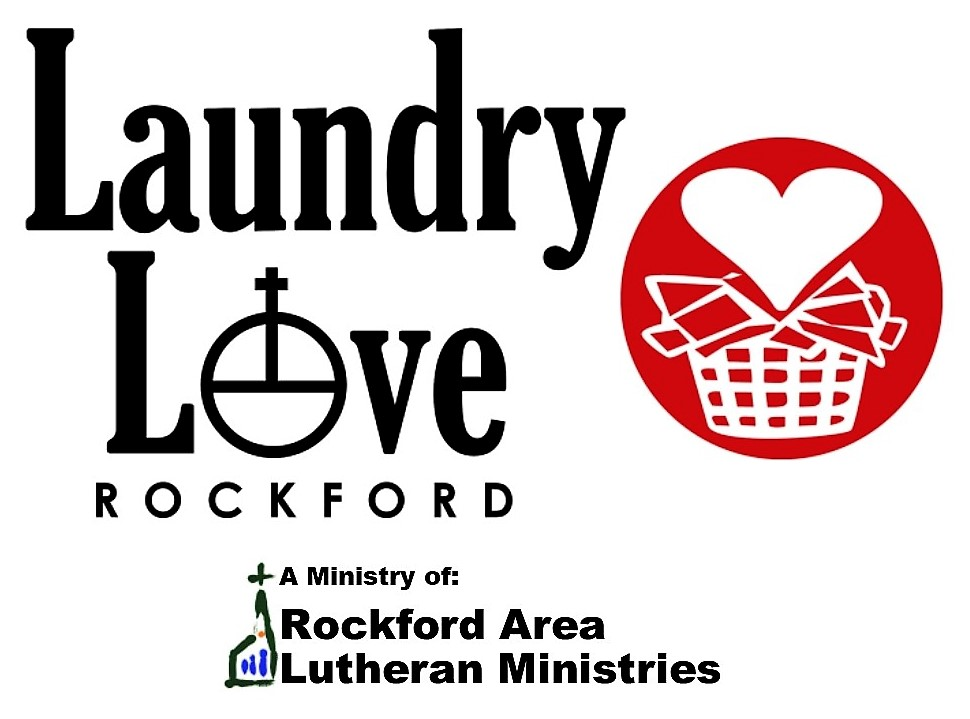Laundry Love Night
On Wednesday, May 15th, St. Mark will sponsor Laundry Love’s monthly open laundry night. In addition to seeking donations the Sunday before, we will also provide the volunteers for the evening. This busy night begins at 5:30 p.m. and ends roughly between 7:30 and 8:00 p.m. after the last client has been served. Volunteers will assist clients with soap and quarters and have plenty of time to talk with those who are there. It is truly a rewarding experience. If you want to participate, please contact Pastor Chad prmckenna@stmarklc.com Space is limited! Laundry Love happens at Charles Street Laundromat (2125 Charles St.) and is a ministry of Rockford Area Lutheran Ministries.

 table div table+table+table+table+table+table+table+table+table+table+table+table+table+table+table+table+table+table+table+table div table{width:100%;padding:0}table div table+table+table+table+table+table+table+table+table+table+table+table+table+table+table+table+table+table+table+table div table img{width:96.23%;padding:0;float:none}table div table+table+table+table+table+table+table+table+table+table+table+table+table+table+table+table+table+table+table+table div table td{width:100%;padding:0 1.88% 18px}/* styles */Family Kite Flying Saturday!
Wind Swept Saturday, May 11, we are hosting a family Kite Flying event at 10:00 A.M. Join us at the Saturn Park for a morning of kite flying and field clean-up. We will celebrate spring and help to make the neighborhood more beautiful! Saturn Park is located at the corner of Garrett Lane and Trainer Road, across from the fire station behind Target. Kites will be provided. Stay tuned for more information.

 table div table+table+table+table+table+table+table+table+table+table+table+table+table+table+table+table+table+table+table+table+table+table div table{width:100%;padding:0}table div table+table+table+table+table+table+table+table+table+table+table+table+table+table+table+table+table+table+table+table+table+table div table img{width:96.23%;padding:0;float:none}table div table+table+table+table+table+table+table+table+table+table+table+table+table+table+table+table+table+table+table+table+table+table div table td{width:100%;padding:0 1.88% 18px}/* styles */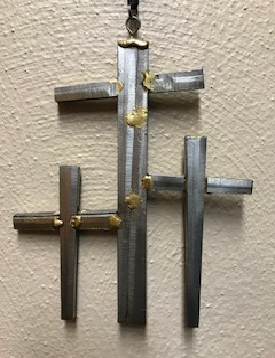We have more of the crosses available for purchase in Fellowship Hall. Please see the display if you are interested. You may also contact Melinda Alekna if you are wanting to purchase a cross. They are just \$10! Proceeds will benefit the youth summer high adventure trip.

 table div table+table+table+table+table+table+table+table+table+table+table+table+table+table+table+table+table+table+table+table+table+table+table+table div table{width:100%;padding:0}table div table+table+table+table+table+table+table+table+table+table+table+table+table+table+table+table+table+table+table+table+table+table+table+table div table img{width:96.23%;padding:0;float:none}table div table+table+table+table+table+table+table+table+table+table+table+table+table+table+table+table+table+table+table+table+table+table+table+table div table td{width:100%;padding:0 1.88% 18px}/* styles */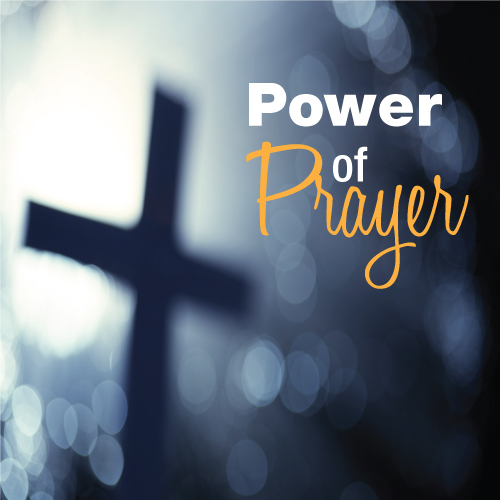St. Mark Prayer Chain
Would you like to be a part of the St. Mark prayer chain? Prayer requests come into the church office weekly. As we receive prayer requests we would send out an e-mail and ask that you pray daily for the persons mentioned in the requests. All requests are to remain confidential. Please e-mail melinda@stmarklc.com if you would like to participate in the prayer chain. “Be anxious for nothing, but in everything by prayer and supplication, with thanksgiving, let your requests be made known to God.” Colossians 4:2.

 table div table+table+table+table+table+table+table+table+table+table+table+table+table+table+table+table+table+table+table+table+table+table+table+table+table+table div table{width:100%;padding:0}table div table+table+table+table+table+table+table+table+table+table+table+table+table+table+table+table+table+table+table+table+table+table+table+table+table+table div table img{width:96.23%;padding:0;float:none}table div table+table+table+table+table+table+table+table+table+table+table+table+table+table+table+table+table+table+table+table+table+table+table+table+table+table div table td{width:100%;padding:0 1.88% 18px}/* styles */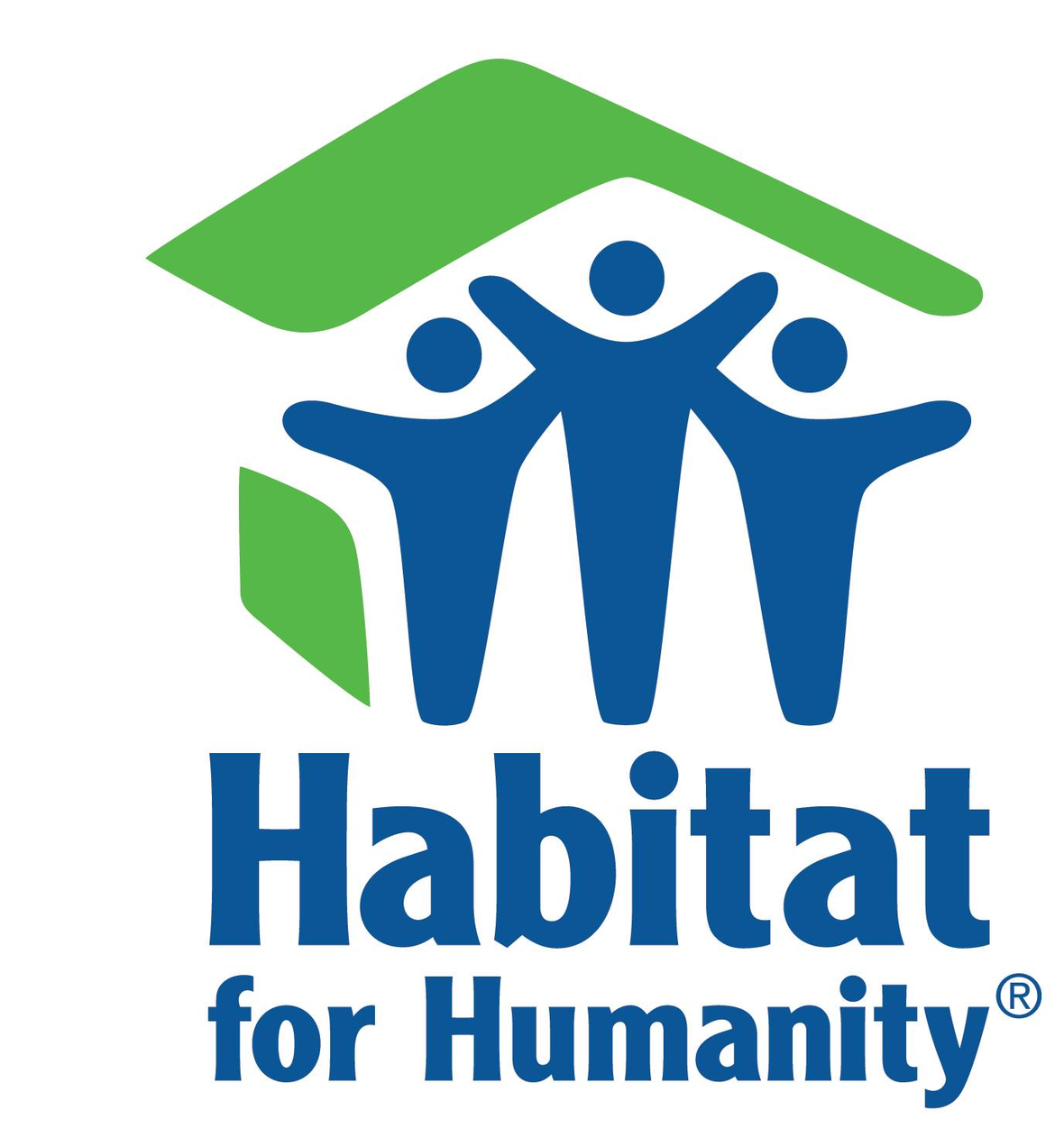Habitat for Humanity Framing Days
Our local Habitat chapter is kicking off the build season on Saturday, May 4th, with their first of four framing days. No matter how handy you are with a hammer, your help is always welcome! To sign up, please go to rockfordhabitat.org/events.

 table div table+table+table+table+table+table+table+table+table+table+table+table+table+table+table+table+table+table+table+table+table+table+table+table+table+table+table+table div table{width:100%;padding:0}table div table+table+table+table+table+table+table+table+table+table+table+table+table+table+table+table+table+table+table+table+table+table+table+table+table+table+table+table div table img{width:96.23%;padding:0;float:none}table div table+table+table+table+table+table+table+table+table+table+table+table+table+table+table+table+table+table+table+table+table+table+table+table+table+table+table+table div table td{width:100%;padding:0 1.88% 18px}/* styles */## Youth MinistrySummer Youth Trip to the Apostle Islands is June 24-28. We have a couple of seats left in the van. We hope to sign-up a couple more youth in March. If you have questions or are interested please email or call Melinda Alekna melinda@stmarklc.com or 815-871-0390.

 table div table+table+table+table+table+table+table+table+table+table+table+table+table+table+table+table+table+table+table+table+table+table+table+table+table+table+table+table+table+table+table div table{width:100%;padding:0}table div table+table+table+table+table+table+table+table+table+table+table+table+table+table+table+table+table+table+table+table+table+table+table+table+table+table+table+table+table+table+table div table img{width:96.23%;padding:0;float:none}table div table+table+table+table+table+table+table+table+table+table+table+table+table+table+table+table+table+table+table+table+table+table+table+table+table+table+table+table+table+table+table div table td{width:100%;padding:0 1.88% 18px}/* styles */## Children's MinistryMay 5 is the last day of Sunday School. We will also recognize our graduating sixth graders. Attendance awards and Sunday school teacher recognition are May 12 at the 9:30 service.

 table div table+table+table+table+table+table+table+table+table+table+table+table+table+table+table+table+table+table+table+table+table+table+table+table+table+table+table+table+table+table+table+table+table+table div table{width:100%;padding:0}table div table+table+table+table+table+table+table+table+table+table+table+table+table+table+table+table+table+table+table+table+table+table+table+table+table+table+table+table+table+table+table+table+table+table div table img{width:96.23%;padding:0;float:none}table div table+table+table+table+table+table+table+table+table+table+table+table+table+table+table+table+table+table+table+table+table+table+table+table+table+table+table+table+table+table+table+table+table+table div table td{width:100%;padding:0 1.88% 18px}/* styles */## Outreach Ministry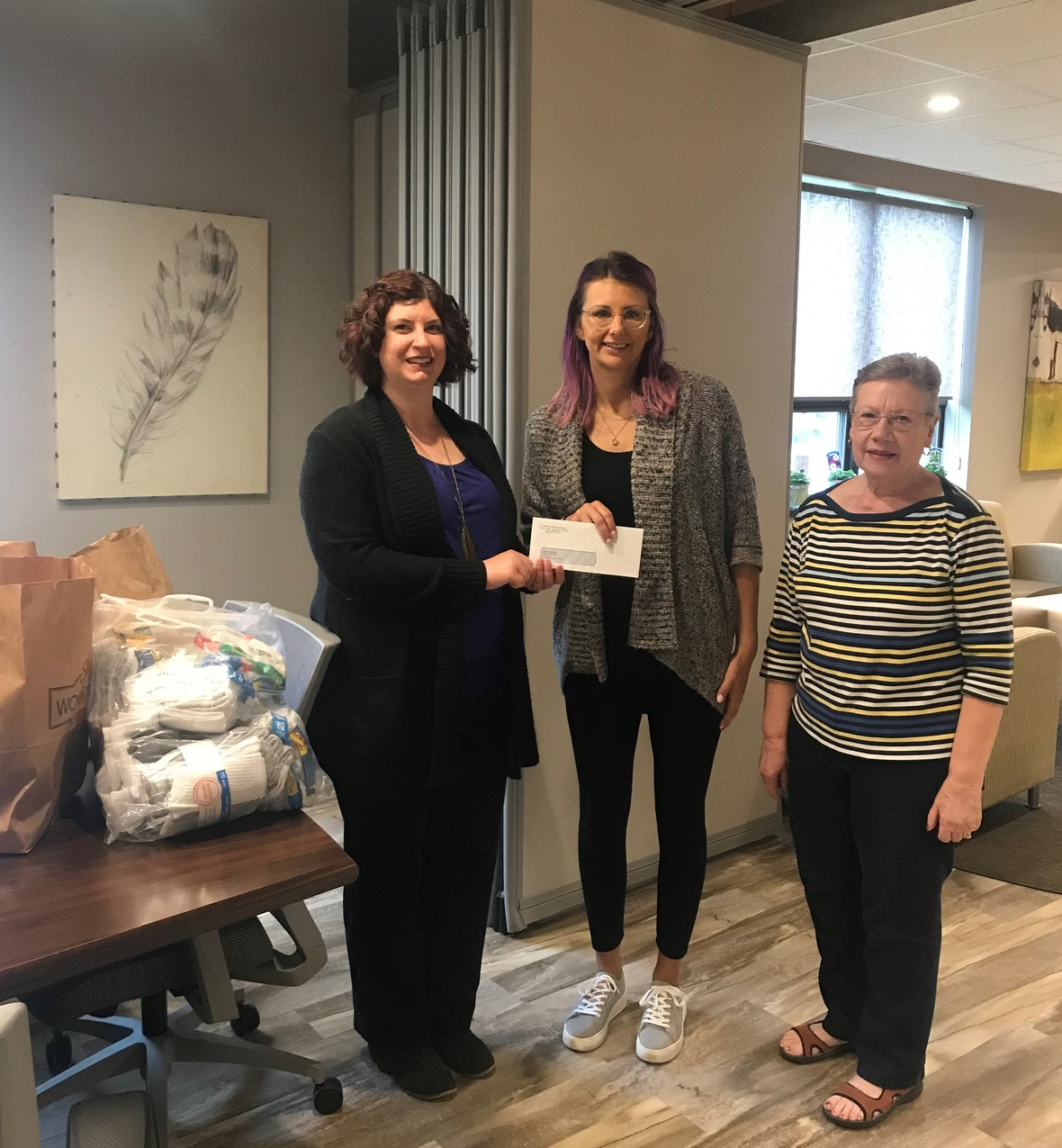Thank You!
During Lent, the Outreach Committee lead the collection of socks to be donated to Project Undershare. Sunday School children also set aside their Lenten offering for Project Undershare.

Together, St. Mark was able to give 318 pairs of new socks and \$150 to be used for the purchase of new underwear, all of which will go to area kids. Thank you to everyone who helped to meet this vital need in our community!

 table div table+table+table+table+table+table+table+table+table+table+table+table+table+table+table+table+table+table+table+table+table+table+table+table+table+table+table+table+table+table+table+table+table+table+table+table+table div table,table.module-36{width:3.77%;float:left;padding:0}table div table+table+table+table+table+table+table+table+table+table+table+table+table+table+table+table+table+table+table+table+table+table+table+table+table+table+table+table+table+table+table+table+table+table+table+table+table div table a{border:0 none;text-decoration:none}table div table+table+table+table+table+table+table+table+table+table+table+table+table+table+table+table+table+table+table+table+table+table+table+table+table+table+table+table+table+table+table+table+table+table+table+table+table div table img{width:100%!important;border:0 none;text-decoration:none}table div table+table+table+table+table+table+table+table+table+table+table+table+table+table+table+table+table+table+table+table+table+table+table+table+table+table+table+table+table+table+table+table+table+table+table+table+table div table td{width:100%;padding:0 20px 20px 0}/* styles */ On April 25th the following St. Mark volunteers prepared and served tuna noodle casserole, salad, cookies & milk to about 60 folks down at Carpenters Place. Outreach thanks - Diane Starbuck, Gretchen Thornbloom, Carol Malmgren, Christy & Mark Malenchik! St. Mark volunteers serve a good noon meal at Carpenters Place every other month.
 table div table+table+table+table+table+table+table+table+table+table+table+table+table+table+table+table+table+table+table+table+table+table+table+table+table+table+table+table+table+table+table+table+table+table+table+table+table+table div table{width:100%;padding:0}table div table+table+table+table+table+table+table+table+table+table+table+table+table+table+table+table+table+table+table+table+table+table+table+table+table+table+table+table+table+table+table+table+table+table+table+table+table+table div table img{width:96.23%;padding:0;float:none}table div table+table+table+table+table+table+table+table+table+table+table+table+table+table+table+table+table+table+table+table+table+table+table+table+table+table+table+table+table+table+table+table+table+table+table+table+table+table div table td{width:100%;padding:0 1.88% 18px}/* styles */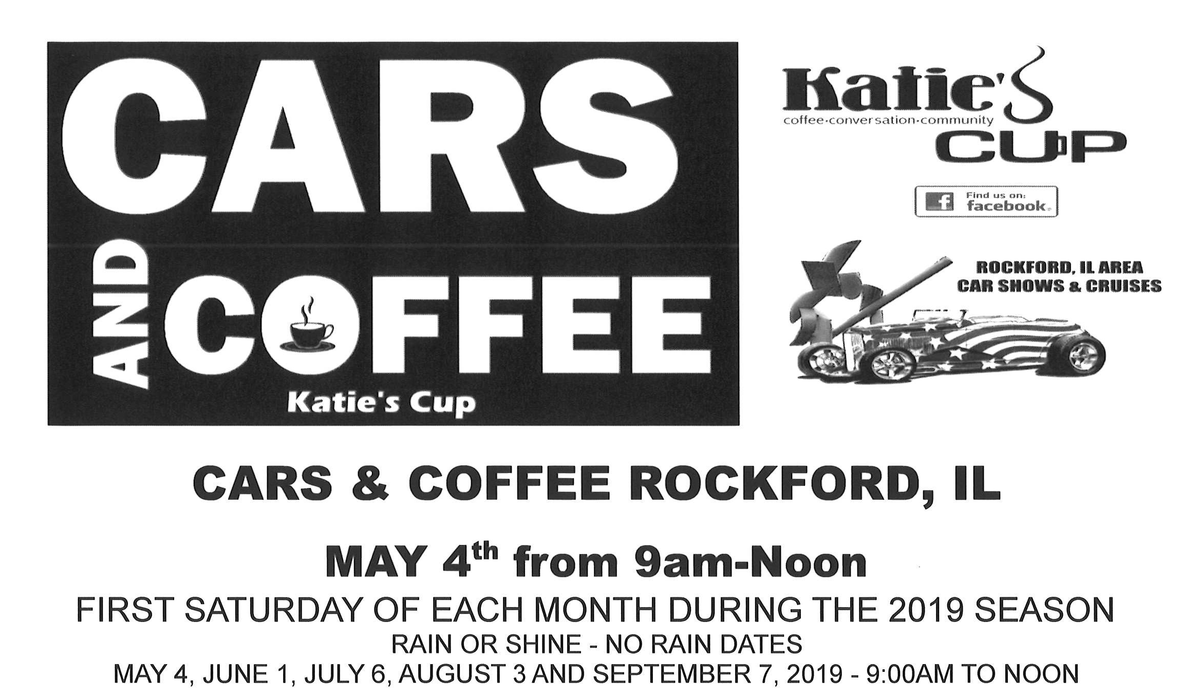table div table+table+table+table+table+table+table+table+table+table+table+table+table+table+table+table+table+table+table+table+table+table+table+table+table+table+table+table+table+table+table+table+table+table+table+table+table+table+table+table+table div table{width:100%;padding:0}table div table+table+table+table+table+table+table+table+table+table+table+table+table+table+table+table+table+table+table+table+table+table+table+table+table+table+table+table+table+table+table+table+table+table+table+table+table+table+table+table+table div table img{width:96.23%;padding:0;float:none}table div table+table+table+table+table+table+table+table+table+table+table+table+table+table+table+table+table+table+table+table+table+table+table+table+table+table+table+table+table+table+table+table+table+table+table+table+table+table+table+table+table div table td{width:100%;padding:0 1.88% 18px}/* styles */## Our St. Mark members:

Deb Lindley, Kim Edgcomb, David Young, Russ Oellig, Linda Swanson, Pam Cottrill, Keith Haggestad, Naomi Hagen.

## Our condolences to:

Bev Berke, Rod & Jodi Bjerke and family upon the death of Bev's husband and Ron's father, Rodney Bjerke.

## Our condolences to:

Aaron and Beth Riggins and family upon the death of Beth's mother, Eldora Watson.

## Family and friends of St. Mark members:

Ann Eck, Clay Heinrich, Denise Nichols, Mary Graham, Doug Nelson, Barbara, Heckart, Tom Wolfe, Kim Menke, Noah and Ellie Adams, Norma Comstock, Scott Jones, Ethan Whitney, Carolyn King, Tom Severson, Katie Butera, Ashton Henley, Nathan Gyllin.

## Those serving in the military and their families:

Bailey Panjkovich, Xander Gehrke, Jim Tammen, Brandon Ballenger, Taylor Eversole, Dean Barron.

 table div table+table+table+table+table+table+table+table+table+table+table+table+table+table+table+table+table+table+table+table+table+table+table+table+table+table+table+table+table+table+table+table+table+table+table+table+table+table+table+table+table+table+table+table div table{width:100%;padding:0}table div table+table+table+table+table+table+table+table+table+table+table+table+table+table+table+table+table+table+table+table+table+table+table+table+table+table+table+table+table+table+table+table+table+table+table+table+table+table+table+table+table+table+table+table div table img{width:96.23%;padding:0;float:none}table div table+table+table+table+table+table+table+table+table+table+table+table+table+table+table+table+table+table+table+table+table+table+table+table+table+table+table+table+table+table+table+table+table+table+table+table+table+table+table+table+table+table+table+table div table td{width:100%;padding:0 1.88% 18px}/* styles *//* styles */ Office Hours Monday - Friday, 8:00 A.M. - 4:00 P.M. Telephone: 815-398-3557 Publications St. Mark Memos, the weekly e-newsletter, is distributed each Thursday. The deadline for submission is Monday by 4:00 P.M. Please click to send submissions. Sermons Online Miss a Sunday at St. Mark? Please visit our website and watch the sermons online. Please click to watch.